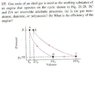# Another Thermo Question

suspenc3

See Attachment

## The Attempt at a Solution

Well to start off I know the gas is monatomic

I can find the work done by finding the area under each curve/line:

$$W_{AB} = p_0(2v_0-v_0) = p_0v_0$$
$$W_{CD} = (p_0/32)(8v_0-16v_0) = \frac{-p_0v_0}{4}$$

I can't think of how to find the area under the other two curves, I am guessing integration but I don't know how to set it up.

After I find these other to, what do I do?

Thanks

#### Attachments

•phys2.jpg
24.5 KB · Views: 343

I can't see the attachment yet, but finding the area between two curves is easy. The easiest way to do it is to subtract the area under the lower curve from the area under the upper curve.

If you did it in one integral, you could set up the limits of integration from one curve to the other.

eaboujaoudeh
for isentropic expansion and compression in ICEs we use the same principle u used to find the first 2 works.
we use : W= (PoVo-PoVo/4)/(1-k) k=Cp/Cv. for isentropic adiabatic compression or expansion.

How did u know the gas was monoatomic?

Homework Helper
for isentropic expansion and compression in ICEs we use the same principle u used to find the first 2 works.
we use : W= (PoVo-PoVo/4)/(1-k) k=Cp/Cv. for isentropic adiabatic compression or expansion.
Where do you get this? It works for BC but not DA

(1) $$W = K\frac{V_f^{1-\gamma} - V_i^{1-\gamma}}{1-\gamma}$$

where $K = PV^\gamma$

This is just the integral $\int dW$ where $dW = dU = PdV = KV^{-\gamma}dV$ (dQ=0)

Since for DA $P_f = 32P_i$ and $V_f = V_i/8$ the numerator in (1) is simply:

$$P_fV_f - P_iV_i = 32P_iV_i/8 - P_iV_i = 3P_iV_i$$

for BC, $P_f = P_i/32$ and $V_f = 8V_i$ the numerator in (1) is simply:

$$P_fV_f - P_iV_i = 8P_iV_i/32 - P_iV_i = -3P_iV_i/4$$

How did u know the gas was monoatomic?

Apply the adiabatic condition $PV^\gamma = constant$ to one of the adiabatic paths and solve for $\gamma$.

AM

Last edited:
suspenc3
Thanks Andrew